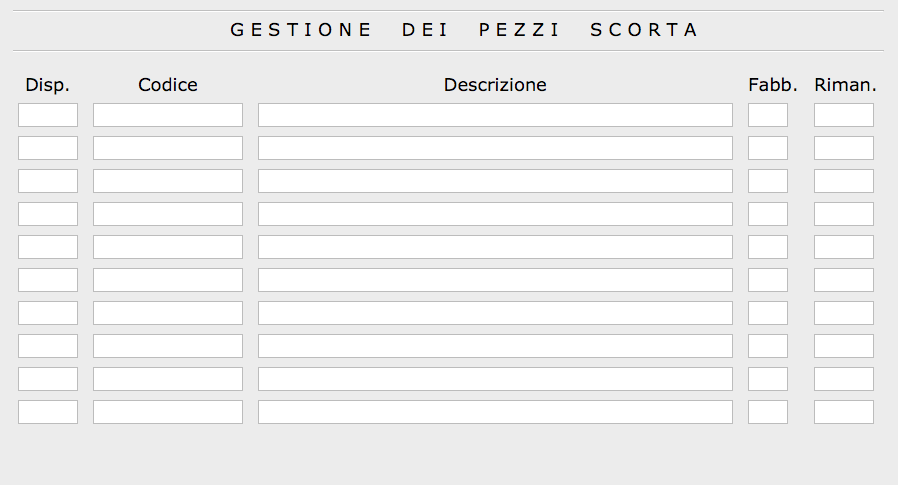# Set Array of QlineEdit to spaces

• Hi,
why when i set an array of QlineEdit to spaces my application crashes??

I need to "clear" array before show new value loaded by loop with a selection in a ComboBox.

this is the code i've used:

``````for  (int i=0; i<10; i++){
array_Cod[i]->setText(" ");
array_Desc[i]->setText(" ");
array_Fabb[i]->setText(" ");
}
``````

if it's not possible how can i clear them??

• Hi,

Are you sure all array elements have been properly allocated before clearing them ?

• @SGaist
All elements was added to form but
some of this can be empty

i've use to show some component who create an article
an article might have 0 min /10 max component

• Then why don't you check that they exists before calling methods on them ?

By the way, you should rather use QVector do store your pointers, you can then more easily properly manage the dynamic side of your widget.

• @SGaist
Ok with check you suggest it works.

one question:
why you tell me to store my pointers using Qvector?
i can't manage it with index of loop like i'm doing now??
what you mean with "more easily properly"?

Thank you

• That makes three questions ;)

1. Because you have a dynamic number of widgets so rather than burning CPU for checking empty widgets, you could use only what you need.
2. That would be the same but you would use the QVector size rather than a fixed number that you will but all over your code. You can still set a maximum though.
3. See previous numbers.

• @SGaist
Ok, i understand..
as soon as possible i will try

I hope i will be able do it

Thank you

• Then why don't you check that they exists before calling methods on them ?
By the way, you should rather use QVector do store your pointers, you can then more easily properly manage the dynamic side of your widget.

Sorry, i'm in error.. don't work..

i've used this code:

``````for  (int i=0; i<10; i++){
if (array_Cod[i]->text() != "") {
array_Cod[i]->setText("");
//array_Desc[i]->setText("");
//array_Fabb[i]->setText("");
}
}
``````

but application crash again..

where is the problem??

• where is the problem??

The problem is that your if is completely useless.
You need to check the pointer:

``````if (array_Cod[i]) {

}
``````

You need to learn use pointers: if you declare a pointer variable but don't assign it a valid pointer and then try to use it your app will crash because to pointer is pointing to not allocated memory.

``````int* some_pointer;
std::cout << *some_pointer; // Crash here
``````

• @jsulm

Later (in the code) i've used this code :

``````int x = 0;
if (q.next())
{
do {
for (int j = x; j < 10; j++){
if (q.value(0) == array_Cod[j]->text()) {
array_Disp[j]->setText(QVariant(q.value(1)).toString());
x++;
} else {
if (array_Cod[j]->text() != ""){
array_Disp[j]->setText("0");
}
}
};
} while (q.next());

}
``````

and it works..

Where is the differences??

• @TheCipo76 I don't know. Maybe this time all pointers in array_Cod were valid ("Later (in the code)")?

• @jsulm

this is my code:

``````void regtemciclo::on_comboBox_Codice_currentTextChanged(const QString &arg1)
{
// CODICE SELEZIONATO
{
// Messaggio Errore
return;
}
// Pulizia Dati Articolo
for  (int i=0; i<10; i++){
if (array_Cod[i]->text() != "") {
//array_Cod[i]->setText("0");
array_Desc[i]->setText("0"); // (1) don't work
//array_Fabb[i]->setText("");
}
}

//Carico Dati Articolo da DATABASE
QSqlQuery q;
q.prepare("Select * from ARTICOLI where CODICE = '"+ arg1 +"'");
q.exec();

if (q.next())
{
ui->lineEdit_Conto->setText(q.value(2).toString());
ui->lineEdit_Esp->setText(q.value(4).toString());
ui->lineEdit_Descrizione->setText(q.value(3).toString());
ui->lineEdit_SAP->setText(q.value(5).toString());
}
// Carico Componenti da DATABASE
//QSqlQuery q;
q.prepare("SELECT CODICE, DESCRIZIONE, QTA FROM COMPONENTI WHERE COMPLESSIVO =" + arg1 + " order by CODICE");
if (q.exec())
{
if (q.next()) {
int i = 0;
do
{
array_Cod[i]->setText(q.value(0).toString());
array_Desc[i]->setText(q.value(1).toString());
array_Fabb[i]->setText(q.value(2).toString());
i++;
}
while (q.next());
}
}
q.prepare("Select Codice, Quantità from PZSCORTA where Complessivo = " + arg1 + "order by Codice");
q.exec();
int x = 0;
if (q.next())
{
do {
for (int j = x; j < 10; j++){
if (q.value(0) == array_Cod[j]->text()) {
array_Disp[j]->setText(QVariant(q.value(1)).toString());
x++;
} else {
if (array_Cod[j]->text() != ""){
array_Disp[j]->setText("0"); // (2) work OK
}
}
};
} while (q.next());

}

}
``````

i'm new with c++ and with Qt both
but i can't view any difference.. but (1) don't work and (2) work..

(• @TheCipo76

``````array_Desc[i]->setText("0"); // (1) don't work
array_Disp[j]->setText("0"); // (2) work OK
``````

Come on array_Desc is not the same as array_Disp, right? So, again you have invalid pointers in array_Desc, but in array_Disp all pointers are valid, that's why second works.
Did you actually try what I suggested?
Simply check whether the pointer is valid or not, this is really basic:

``````if (array_Desc[i])
array_Desc[i]->setText("0");
``````

And as already suggested you should use QVector instead of arrays, because array have fixed length.
And if you still prefer to use arrays then you should at least make sure you initialize them with 0 or nullptr at the beginning, so the check I posted can work.

• @jsulm
OK, now works

Thank you